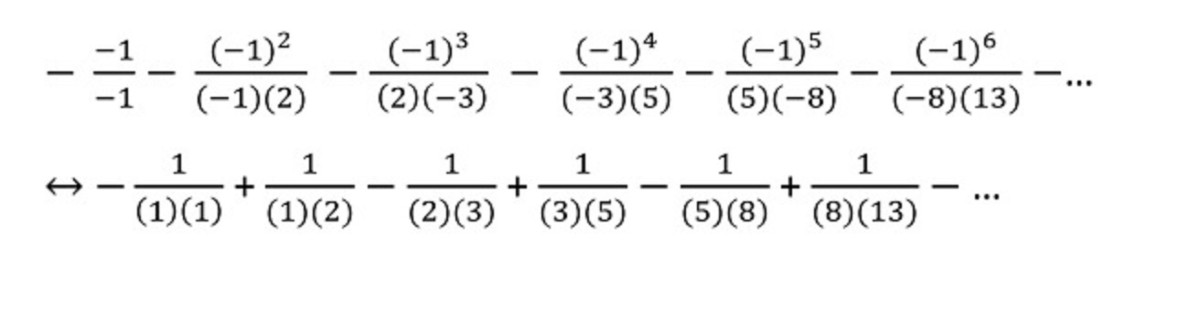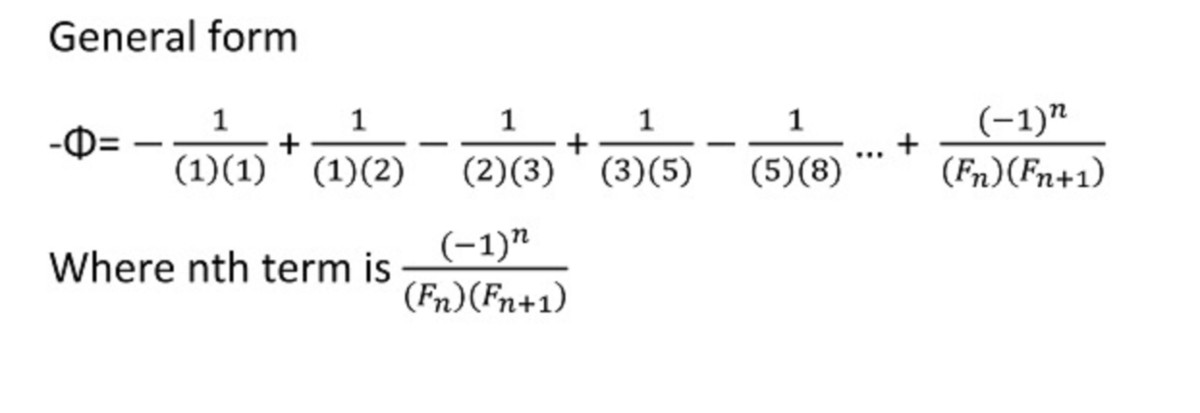# Whittaker Formula and the Fibonacci Numbers

• Author:
• Updated date:

Raul finished his education with a B.S degree in Mathematics and a Minor in Physics. Recently, he became an amateur stargazer.

## A Special Polynomial Equation

This article uses a specific polynomial equation to introduce the Whittaker method for finding the root that has the smallest absolute value. I will use the polynomial x2-x-1=0. This polynomial is special since the roots are x1=ϕ (golden ratio) ≈1.6180 and x2=-Φ ( negative of golden ratio conjugate)≈ - 0.6180.

## Whittaker Formula

Whittaker formula is a method that uses the coefficients of the polynomial equation to create some special matrices. The determinants of these special matrices are used to create an infinite series that converges to the root that has the smallest absolute value. If we have the following general polynomial 0=a0 + a1x+ a2x2 + a3x3+a4x4 + … , the smallest root in absolute value is given by the equation found in image 1. Wherever you see a matrix in image 1, the determinant of that matrix is meant to be in its place.

The formula doesn’t work if there is more than one root with the smallest absolute value. For example, if the smallest roots are 1 and -1, you cannot use the Whittaker formula since abs(1)= abs(-1)=1. This problem can be easily bypassed by transforming the initial polynomial into another polynomial. I will deal with this problem in another article since the polynomial that I will use in this article doesn’t have this problem.

## Specific Example

The smallest root in absolute value of 0= x2-x-1 is x2=-Φ (negative of golden ratio conjugate)≈ - 0.6180. So we must obtain an infinite series that converges to x2. Using the same notation as in the previous section, we get the following assignments a0=-1, a1=-1, and a2=1. If we look at the formula from image 1 we can see that we actually need an infinite number of coefficients and we have only 3 coefficients. All the other coefficients have a value of zero, thus a3=0 , a4=0 , a5=0, etc.

The matrices from the numerator of our terms always start with the element m1,1=a2=1. In image 2 I show the determinants of the 2x2, 3x3, and 4x4 matrix that start with the element m1,1=a2=1. The determinant of these matrices is always 1 since these matrices are lower triangular matrices and the product of the elements from the main diagonal is 1n=1.

Now we should look at the matrices from the denominator of our terms. In the denominator, we always have matrices that start with the element m1,1=a1=-1. In image 3 I show the 2x2,3x3,4x4,5x5 and 6x6 matrices and their determinants. The determinants in the proper order are 2, -3, 5, -8, and 13. So we obtain successive Fibonacci numbers, but the sign alternates between positive and negative. I didn’t bother to find a proof that shows that these matrices indeed generate determinants equal to successive Fibonacci numbers (with alternating signs), but I may try in the future. In image 4 I provide the first few terms in our infinite series. In image 5 I try to generalize the infinite series using the Fibonacci numbers. If we let F1=1, F2=1, and F3=2, then the formula from image 5 should be correct.

Finally, we can use the series from image 5 to generate an infinite series for the golden number. We can use the fact that φ=Φ +1, but we also have to reverse the signs of the terms from image 5 since that is an infinite series for -Φ.

Scroll to Continue

## Read More From OwlcationFirst few terms of the infinite seriesRaulPGeneral formula of the infinite seriesRaulP

## Final Remarks

If you want to learn more about the Whittaker method you should check the source  that I provide at the bottom of this article. I think it is amazing that by using this method you can obtain a sequence of matrices that have determinants with meaningful values. Searching the internet I found the infinite series obtained in this article. This infinite series was mentioned in a forum discussion, but I could not find a more detailed article that discusses this particular infinite series.

You can try to apply this method to other polynomials and you may find other interesting infinite series. In a future article, I will show how to obtain an infinite series for a square root of 2 using the Pell numbers.